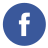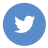### after and before in java date

java

To check if one java.time.LocalDate object is before or after another date, you can use the isBefore() and isAfter() methods.

Here’s an example:

``````import java.time.LocalDate;

public class Main {
public static void main(String[] args) {
LocalDate date1 = LocalDate.of(2020, 1, 1);
LocalDate date2 = LocalDate.of(2020, 1, 2);
LocalDate date3 = LocalDate.of(2020, 1, 1);

System.out.println(date1.isBefore(date2)); // true
System.out.println(date1.isBefore(date3)); // false
System.out.println(date1.isAfter(date2)); // false
System.out.println(date1.isAfter(date3)); // false
}
}``````

You can also use the isEqual() method to check if two dates are equal:

``````import java.time.LocalDate;

public class Main {
public static void main(String[] args) {
LocalDate date1 = LocalDate.of(2020, 1, 1);
LocalDate date2 = LocalDate.of(2020, 1, 2);
LocalDate date3 = LocalDate.of(2020, 1, 1);

System.out.println(date1.isEqual(date2)); // false
System.out.println(date1.isEqual(date3)); // true
}
}``````

You can use these methods with the java.time.LocalDateTime class as well.